CLAT  >  Quant Mock Test - 13

# Quant Mock Test - 13

Test Description

## 20 Questions MCQ Test | Quant Mock Test - 13

Quant Mock Test - 13 for CLAT 2022 is part of CLAT preparation. The Quant Mock Test - 13 questions and answers have been prepared according to the CLAT exam syllabus.The Quant Mock Test - 13 MCQs are made for CLAT 2022 Exam. Find important definitions, questions, notes, meanings, examples, exercises, MCQs and online tests for Quant Mock Test - 13 below.
Solutions of Quant Mock Test - 13 questions in English are available as part of our course for CLAT & Quant Mock Test - 13 solutions in Hindi for CLAT course. Download more important topics, notes, lectures and mock test series for CLAT Exam by signing up for free. Attempt Quant Mock Test - 13 | 20 questions in 30 minutes | Mock test for CLAT preparation | Free important questions MCQ to study for CLAT Exam | Download free PDF with solutions
 1 Crore+ students have signed up on EduRev. Have you?
Quant Mock Test - 13 - Question 1

### If both the radius and height of a right circular cone are increased by 20%, its volume will be increased by

Detailed Solution for Quant Mock Test - 13 - Question 1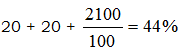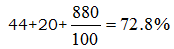Quant Mock Test - 13 - Question 2

### 3 years ago, the average age of family of 5 members was 17 years. A baby having been born, the average age of the family is the same today. The present age of the baby is:

Detailed Solution for Quant Mock Test - 13 - Question 2

3 yr ago, age Sum of Five Member = 85
Therefore present total of five member = 85 + 15 = 100 yr.
After Baby Birth average remain same = 17
∴ 6 Members
Total = 17 x 6 = 102
∴ Baby age = 2 yr.

Quant Mock Test - 13 - Question 3

###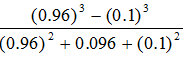is simplified to:

Detailed Solution for Quant Mock Test - 13 - Question 3

(a – b)
= 0.96 – 0.1
= 0.86.

Quant Mock Test - 13 - Question 4

The square root of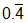is

Detailed Solution for Quant Mock Test - 13 - Question 4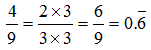Quant Mock Test - 13 - Question 5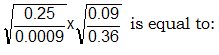Detailed Solution for Quant Mock Test - 13 - Question 5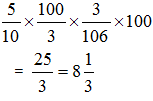Quant Mock Test - 13 - Question 6

The ratio of ages of two students is 3 : 2. One is older to the other by 5 years. What is the age of the younger student?

Quant Mock Test - 13 - Question 7

The HCF of two numbers is 23 and the other two factors of their LCM are 13 and 14. The larger of the two numbers is:

Detailed Solution for Quant Mock Test - 13 - Question 7

Larger Number = 23 x 14
= 322.

Quant Mock Test - 13 - Question 8

The next number in the sequence 2, 8, 18, 32, 50, …… is:

Detailed Solution for Quant Mock Test - 13 - Question 8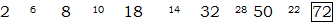Quant Mock Test - 13 - Question 9

Out of 450 students of a school 325 play football, 175 play cricket and 50 neither play football nor cricket, How many students play both football and cricket?

Detailed Solution for Quant Mock Test - 13 - Question 9

The correct answer is B as by drawing vein diagram 100 is the correct answer.

Quant Mock Test - 13 - Question 10

The length of a rectangle is decreased by 10% and its breadth is increased by 10%. By what per cent is its area changed?

Detailed Solution for Quant Mock Test - 13 - Question 10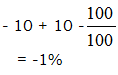Quant Mock Test - 13 - Question 11

A man can row at 5 kmph. in still water. If the velocity of current is 1 kmph and it takes him 1 hour to row to a place and come back, how far is the place?

Detailed Solution for Quant Mock Test - 13 - Question 11

u + v = 6
u – v = 4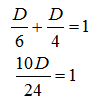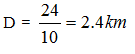Quant Mock Test - 13 - Question 12

The average weight of A, B, C is 45 kg. If the average weight of A and B be 40 kg and that of B and C be 43 kg, then the weight of B is

Detailed Solution for Quant Mock Test - 13 - Question 12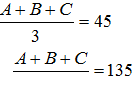80 + C = 135
B = 55
A + B = 80/ B + C = 86
55 + C = 86
C = 31.

Quant Mock Test - 13 - Question 13

A man can do a piece of work in 5 days, but with the help of his son, he can do it in 3 days. In what time can the son do it alone?

Detailed Solution for Quant Mock Test - 13 - Question 13

15 chairs
A = 3 ch
A + B = 5 ch
B = 2 ch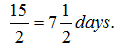Quant Mock Test - 13 - Question 14

Some articles were bought at 6 for Rs. 5, and sold at 5 for Rs. 6. Gain per cent is:

Detailed Solution for Quant Mock Test - 13 - Question 14

C.P = Rs 25
S.P = Rs 36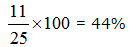Quant Mock Test - 13 - Question 15

Rs. 750 are divided among A, B and C in such a manner that A : B = 5 : 2 and B : C = 7 : 13. What is A’s share?

Detailed Solution for Quant Mock Test - 13 - Question 15

5 : 2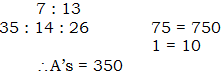Quant Mock Test - 13 - Question 16

A truck covers a distance of 550 metres in 1 minute whereas a bus covers a distance of 33 kms in 45 minutes. The ratio of their speeds is:

Detailed Solution for Quant Mock Test - 13 - Question 16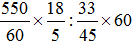3 : 4

Quant Mock Test - 13 - Question 17

If the ratio of areas of two squares is 225:256, then the ratio of their perimeters is:

Detailed Solution for Quant Mock Test - 13 - Question 17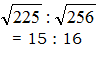Quant Mock Test - 13 - Question 18

If 30% of A = 0.25 of B = 1/5 of C, then A : B : C is equal to:

Detailed Solution for Quant Mock Test - 13 - Question 18

0.30 A = 0.25B = 0.2C
So ratio = 10 : 12 : 15

Quant Mock Test - 13 - Question 19

The percentage increase in the area of a rectangle, if each of its sides is increased by 20% is:

Detailed Solution for Quant Mock Test - 13 - Question 19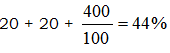Quant Mock Test - 13 - Question 20

A number is increased by 20% and then again by 20%. By what per cent should the increased number be reduced so as to get back the original number?

Detailed Solution for Quant Mock Test - 13 - Question 20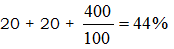Increase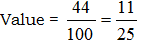Value to be decreased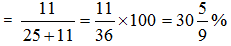Use Code STAYHOME200 and get INR 200 additional OFF Use Coupon Code
Information about Quant Mock Test - 13 Page
In this test you can find the Exam questions for Quant Mock Test - 13 solved & explained in the simplest way possible. Besides giving Questions and answers for Quant Mock Test - 13, EduRev gives you an ample number of Online tests for practice